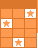Challenge Board: Score points by answering questions correctly.# ALGEBRAIC FRACTIONS

To simplify, multiply, divide, add and subtract algebraic fractions by factoring first.This activity was created by a Quia Web subscriber. Learn more about QuiaCreate your own activities